# 从零开始PyTorch项目：YOLO v3目标检测实现

2019/12/07 08:19

https://github.com/ayooshkathuria/YOLO_v3_tutorial_from_scratch

1. YOLO 的工作原理

2. 创建 YOLO 网络层级

3. 实现网络的前向传播

4. objectness 置信度阈值和非极大值抑制

5. 设计输入和输出管道

• 卷积神经网络的工作原理，包括残差块、跳过连接和上采样；

• 目标检测、边界框回归、IoU 和非极大值抑制；

• 基础的 PyTorch 使用。你需要能够轻松地创建简单的神经网络。

YOLO 是 You Only Look Once 的缩写。它是一种使用深度卷积神经网络学得的特征来检测对象的目标检测器。在我们上手写代码之前，我们必须先了解 YOLO 的工作原理。

YOLO 仅使用卷积层，这就使其成为全卷积神经网络（FCN）。它拥有 75 个卷积层，还有跳过连接和上采样层。它不使用任何形式的池化，使用步幅为 2 的卷积层对特征图进行下采样。这有助于防止通常由池化导致的低级特征丢失。

YOLO 通过被步幅对图像进行上采样。例如，如果网络的步幅是 32，则大小为 416×416 的输入图像将产生 13×13 的输出。通常，网络层中的任意步幅都指层的输入除以输入。

1×1×（B×（5+C））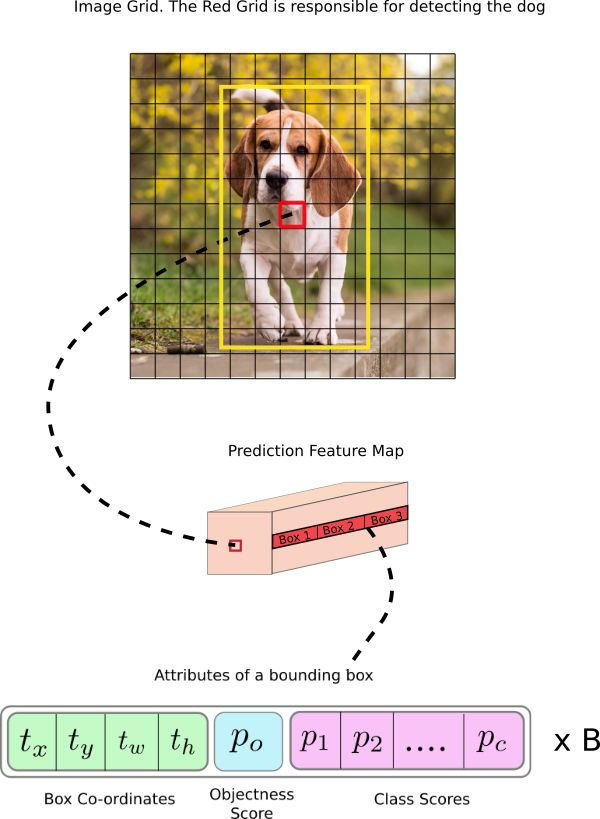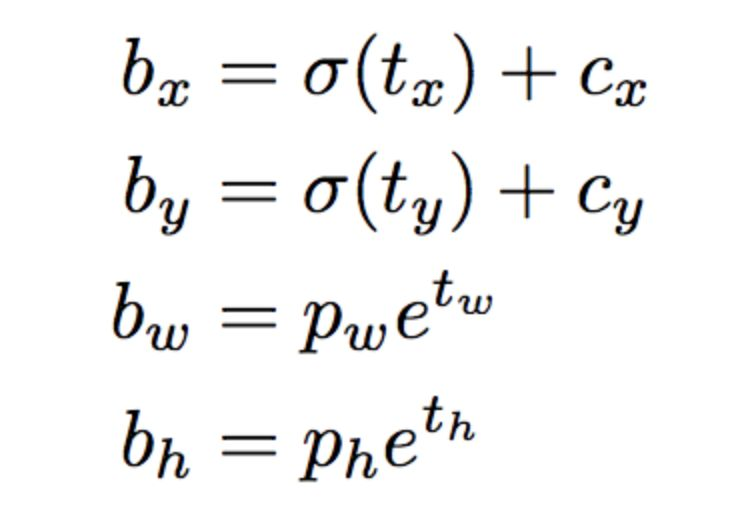• 与预测目标的网格单元左上角相关的偏移；

• 使用特征图单元的维度（1）进行归一化的偏移。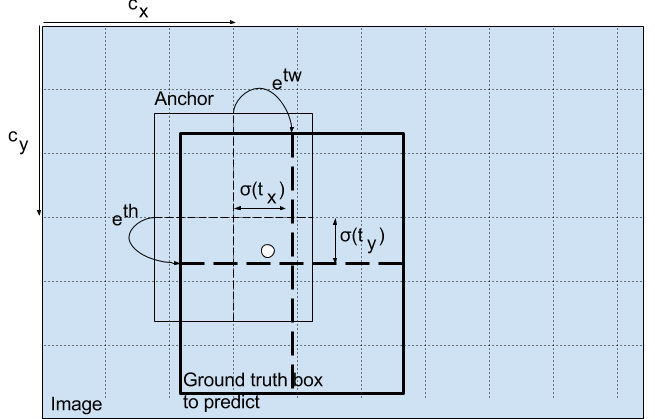Objectness 分数

Object 分数表示目标在边界框内的概率。红色网格和相邻网格的 Object 分数应该接近 1，而角落处的网格的 Object 分数可能接近 0。

objectness 分数的计算也使用 sigmoid 函数，因此它可以被理解为概率。

YOLO v3 在 3 个不同尺度上进行预测。检测层用于在三个不同大小的特征图上执行预测，特征图步幅分别是 32、16、8。这意味着，当输入图像大小是 416 x 416 时，我们在尺度 13 x 13、26 x 26 和 52 x 52 上执行检测。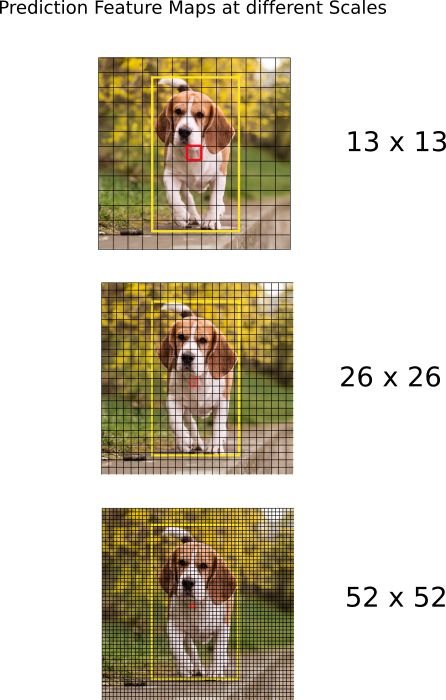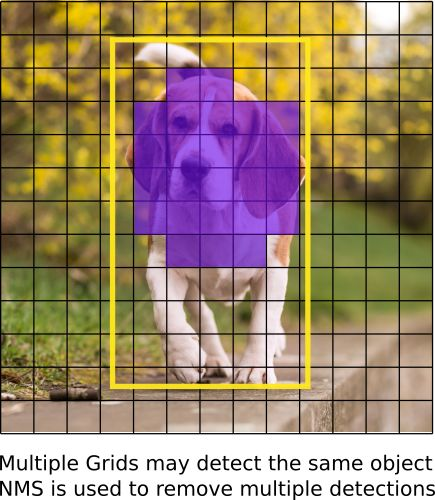YOLO 只能检测出属于训练所用数据集中类别的对象。我们的检测器将使用官方权重文件，这些权重通过在 COCO 数据集上训练网络而获得，因此我们可以检测 80 个对象类别。

1. YOLO V1: You Only Look Once: Unified, Real-Time Object Detection (https://arxiv.org/pdf/1506.02640.pdf)

2. YOLO V2: YOLO9000: Better, Faster, Stronger (https://arxiv.org/pdf/1612.08242.pdf)

3. YOLO V3: An Incremental Improvement (https://pjreddie.com/media/files/papers/YOLOv3.pdf)

4. Convolutional Neural Networks (http://cs231n.github.io/convolutional-networks/)

5. Bounding Box Regression (Appendix C) (https://arxiv.org/pdf/1311.2524.pdf)

8. PyTorch Official Tutorial (http://pytorch.org/tutorials/beginner/deep_learning_60min_blitz.html)

mkdir cfgcd cfgwget https://raw.githubusercontent.com/pjreddie/darknet/master/cfg/yolov3.cfg

[convolutional]batch_normalize=1filters=64size=3stride=2pad=1activation=leaky[convolutional]batch_normalize=1filters=32size=1stride=1pad=1activation=leaky[convolutional]batch_normalize=1filters=64size=3stride=1pad=1activation=leaky[shortcut]from=-3activation=linear

1. 卷积层

[convolutional]batch_normalize=1 filters=64 size=3 stride=1 pad=1 activation=leaky

2. 跳过连接

[shortcut]from=-3 activation=linear 

3.上采样

[upsample]stride=2

4.路由层（Route）

[route]layers = -4[route]layers = -1, 61

5.YOLO

[yolo]mask = 0,1,2anchors = 10,13, 16,30, 33,23, 30,61, 62,45, 59,119, 116,90, 156,198, 373,326classes=80num=9jitter=.3ignore_thresh = .5truth_thresh = 1random=1

YOLO 层级对应于上文所描述的检测层级。参数 anchors 定义了 9 组锚点，但是它们只是由 mask 标签使用的属性所索引的锚点。这里，mask 的值为 0、1、2 表示了第一个、第二个和第三个使用的锚点。而掩码表示检测层中的每一个单元预测三个框。总而言之，我们检测层的规模为 3，并装配总共 9 个锚点。

Net

[net]# Testingbatch=1subdivisions=1# Training# batch=64# subdivisions=16width= 320height = 320channels=3momentum=0.9decay=0.0005angle=0saturation = 1.5exposure = 1.5hue=.1

from __future__ import divisionimport torch import torch.nn as nnimport torch.nn.functional as F from torch.autograd import Variableimport numpy as np

def parse_cfg(cfgfile): """ Takes a configuration file Returns a list of blocks. Each blocks describes a block in the neural network to be built. Block is represented as a dictionary in the list """

file = open(cfgfile, 'r')lines = file.read().split('\n') # store the lines in a listlines = [x for x in lines if len(x) > 0] # get read of the empty lines lines = [x for x in lines if x != '#'] # get rid of commentslines = [x.rstrip().lstrip() for x in lines] # get rid of fringe whitespaces

block = {}blocks = []for line in lines: if line == "[": # This marks the start of a new block if len(block) != 0: # If block is not empty, implies it is storing values of previous block. blocks.append(block) # add it the blocks list block = {} # re-init the block block["type"] = line[1:-1].rstrip()  else: key,value = line.split("=")  block[key.rstrip()] = value.lstrip()blocks.append(block)return blocks

create_modules 函数使用 parse_cfg 函数返回的 blocks 列表：

def create_modules(blocks): net_info = blocks #Captures the information about the input and pre-processing  module_list = nn.ModuleList() prev_filters = 3 output_filters = []

nn.ModuleList

 for index, x in enumerate(blocks[1:]): module = nn.Sequential() #check the type of block #create a new module for the block #append to module_list

nn.Sequential 类被用于按顺序地执行 nn.Module 对象的一个数字。如果你查看 cfg 文件，你会发现，一个模块可能包含多于一个层。例如，一个 convolutional 类型的模块有一个批量归一化层、一个 leaky ReLU 激活层以及一个卷积层。我们使用 nn.Sequential 将这些层串联起来，得到 add_module 函数。例如，以下展示了我们如何创建卷积层和上采样层的例子：

 if (x["type"] == "convolutional"): #Get the info about the layer activation = x["activation"] try: batch_normalize = int(x["batch_normalize"]) bias = False except: batch_normalize = 0 bias = True filters= int(x["filters"]) padding = int(x["pad"]) kernel_size = int(x["size"]) stride = int(x["stride"]) if padding: pad = (kernel_size - 1) // 2 else: pad = 0 #Add the convolutional layer conv = nn.Conv2d(prev_filters, filters, kernel_size, stride, pad, bias = bias) module.add_module("conv_{0}".format(index), conv) #Add the Batch Norm Layer if batch_normalize: bn = nn.BatchNorm2d(filters) module.add_module("batch_norm_{0}".format(index), bn) #Check the activation.  #It is either Linear or a Leaky ReLU for YOLO if activation == "leaky": activn = nn.LeakyReLU(0.1, inplace = True) module.add_module("leaky_{0}".format(index), activn) #If it's an upsampling layer #We use Bilinear2dUpsampling elif (x["type"] == "upsample"): stride = int(x["stride"]) upsample = nn.Upsample(scale_factor = 2, mode = "bilinear") module.add_module("upsample_{}".format(index), upsample)

 #If it is a route layer elif (x["type"] == "route"): x["layers"] = x["layers"].split(',') #Start of a route start = int(x["layers"]) #end, if there exists one. try: end = int(x["layers"]) except: end = 0 #Positive anotation if start > 0:  start = start - index if end > 0: end = end - index route = EmptyLayer() module.add_module("route_{0}".format(index), route) if end < 0: filters = output_filters[index + start] + output_filters[index + end] else: filters= output_filters[index + start] #shortcut corresponds to skip connection elif x["type"] == "shortcut": shortcut = EmptyLayer() module.add_module("shortcut_{}".format(index), shortcut)

route = EmptyLayer()

class EmptyLayer(nn.Module): def __init__(self): super(EmptyLayer, self).__init__()

if end < 0: #If we are concatenating maps filters = output_filters[index + start] + output_filters[index + end]else: filters= output_filters[index + start]

YOLO 层

 #Yolo is the detection layer elif x["type"] == "yolo": mask = x["mask"].split(",") mask = [int(x) for x in mask] anchors = x["anchors"].split(",") anchors = [int(a) for a in anchors] anchors = [(anchors[i], anchors[i+1]) for i in range(0, len(anchors),2)] anchors = [anchors[i] for i in mask] detection = DetectionLayer(anchors) module.add_module("Detection_{}".format(index), detection)

class DetectionLayer(nn.Module): def __init__(self, anchors): super(DetectionLayer, self).__init__() self.anchors = anchors

 module_list.append(module) prev_filters = filters output_filters.append(filters)

return (net_info, module_list)

blocks = parse_cfg("cfg/yolov3.cfg")print(create_modules(blocks))

 (9): Sequential( (conv_9): Conv2d (128, 64, kernel_size=(1, 1), stride=(1, 1), bias=False) (batch_norm_9): BatchNorm2d(64, eps=1e-05, momentum=0.1, affine=True) (leaky_9): LeakyReLU(0.1, inplace) ) (10): Sequential( (conv_10): Conv2d (64, 128, kernel_size=(3, 3), stride=(1, 1), padding=(1, 1), bias=False) (batch_norm_10): BatchNorm2d(128, eps=1e-05, momentum=0.1, affine=True) (leaky_10): LeakyReLU(0.1, inplace) ) (11): Sequential( (shortcut_11): EmptyLayer( ) )

• 阅读本教程前两部分；

• PyTorch 基础知识，包括如何使用 nn.Module、nn.Sequential 和 torch.nn.parameter 创建自定义架构；

• 在 PyTorch 中处理图像。

class Darknet(nn.Module): def __init__(self, cfgfile): super(Darknet, self).__init__() self.blocks = parse_cfg(cfgfile) self.net_info, self.module_list = create_modules(self.blocks)

forward 主要有两个目的。一，计算输出；二，尽早处理的方式转换输出检测特征图（例如转换之后，这些不同尺度的检测图就能够串联，不然会因为不同维度不可能实现串联）。

def forward(self, x, CUDA): modules = self.blocks[1:] outputs = {} #We cache the outputs for the route layer

forward 函数有三个参数：self、输入 x 和 CUDA（如果是 true，则使用 GPU 来加速前向传播）。

write = 0 #This is explained a bit laterfor i, module in enumerate(modules):  module_type = (module["type"])

 if module_type == "convolutional" or module_type == "upsample": x = self.module_list[i](x)

 elif module_type == "route": layers = module["layers"] layers = [int(a) for a in layers] if (layers) > 0: layers = layers - i if len(layers) == 1: x = outputs[i + (layers)] else: if (layers) > 0: layers = layers - i map1 = outputs[i + layers] map2 = outputs[i + layers] x = torch.cat((map1, map2), 1) elif module_type == "shortcut": from_ = int(module["from"]) x = outputs[i-1] + outputs[i+from_]

YOLO（检测层）

YOLO 的输出是一个卷积特征图，包含沿特征图深度的边界框属性。边界框属性由彼此堆叠的单元格预测得出。因此，如果你需要在 (5,6) 处访问单元格的第二个边框，那么你需要通过 map[5,6, (5+C): 2*(5+C)] 将其编入索引。这种格式对于输出处理过程（例如通过目标置信度进行阈值处理、添加对中心的网格偏移、应用锚点等）很不方便。

from __future__ import divisionimport torch import torch.nn as nnimport torch.nn.functional as F from torch.autograd import Variableimport numpy as npimport cv2 

predict_transform 使用 5 个参数：prediction（我们的输出）、inp_dim（输入图像的维度）、anchors、num_classes、CUDA flag（可选）。

def predict_transform(prediction, inp_dim, anchors, num_classes, CUDA = True):

predict_transform 函数把检测特征图转换成二维张量，张量的每一行对应边界框的属性，如下所示：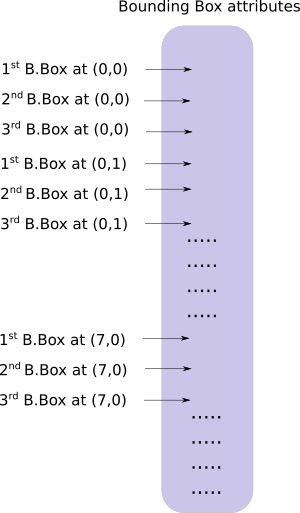batch_size = prediction.size(0) stride = inp_dim // prediction.size(2) grid_size = inp_dim // stride bbox_attrs = 5 + num_classes num_anchors = len(anchors) prediction = prediction.view(batch_size, bbox_attrs*num_anchors, grid_size*grid_size) prediction = prediction.transpose(1,2).contiguous() prediction = prediction.view(batch_size, grid_size*grid_size*num_anchors, bbox_attrs)

 anchors = [(a/stride, a/stride) for a in anchors]

 #Sigmoid the centre_X, centre_Y. and object confidencce prediction[:,:,0] = torch.sigmoid(prediction[:,:,0]) prediction[:,:,1] = torch.sigmoid(prediction[:,:,1]) prediction[:,:,4] = torch.sigmoid(prediction[:,:,4])

 #Add the center offsets grid = np.arange(grid_size) a,b = np.meshgrid(grid, grid) x_offset = torch.FloatTensor(a).view(-1,1) y_offset = torch.FloatTensor(b).view(-1,1) if CUDA: x_offset = x_offset.cuda() y_offset = y_offset.cuda() x_y_offset = torch.cat((x_offset, y_offset), 1).repeat(1,num_anchors).view(-1,2).unsqueeze(0) prediction[:,:,:2] += x_y_offset

 #log space transform height and the width anchors = torch.FloatTensor(anchors) if CUDA: anchors = anchors.cuda() anchors = anchors.repeat(grid_size*grid_size, 1).unsqueeze(0) prediction[:,:,2:4] = torch.exp(prediction[:,:,2:4])*anchors

 prediction[:,:,5: 5 + num_classes] = torch.sigmoid((prediction[:,:, 5 : 5 + num_classes]))

prediction[:,:,:4] *= stride

loop 部分到这里就大致结束了。

 return prediction

from util import * 

 elif module_type == 'yolo':  anchors = self.module_list[i].anchors #Get the input dimensions inp_dim = int (self.net_info["height"]) #Get the number of classes num_classes = int (module["classes"]) #Transform  x = x.data x = predict_transform(x, inp_dim, anchors, num_classes, CUDA) if not write: #if no collector has been intialised.  detections = x write = 1 else:  detections = torch.cat((detections, x), 1) outputs[i] = x

 return detections

wget https://github.com/ayooshkathuria/pytorch-yolo-v3/raw/master/dog-cycle-car.png

def get_test_input(): img = cv2.imread("dog-cycle-car.png") img = cv2.resize(img, (416,416)) #Resize to the input dimension img_ = img[:,:,::-1].transpose((2,0,1)) # BGR -> RGB | H X W C -> C X H X W  img_ = img_[np.newaxis,:,:,:]/255.0 #Add a channel at 0 (for batch) | Normalise img_ = torch.from_numpy(img_).float() #Convert to float img_ = Variable(img_) # Convert to Variable return img_

model = Darknet("cfg/yolov3.cfg")inp = get_test_input()pred = model(inp)print (pred)

( 0 ,.,.) =  16.0962 17.0541 91.5104 ... 0.4336 0.4692 0.5279 15.1363 15.2568 166.0840 ... 0.5561 0.5414 0.5318 14.4763 18.5405 409.4371 ... 0.5908 0.5353 0.4979 ⋱ ...  411.2625 412.0660 9.0127 ... 0.5054 0.4662 0.5043 412.1762 412.4936 16.0449 ... 0.4815 0.4979 0.4582 412.1629 411.4338 34.9027 ... 0.4306 0.5462 0.4138[torch.FloatTensor of size 1x10647x85]

wget https://pjreddie.com/media/files/yolov3.weights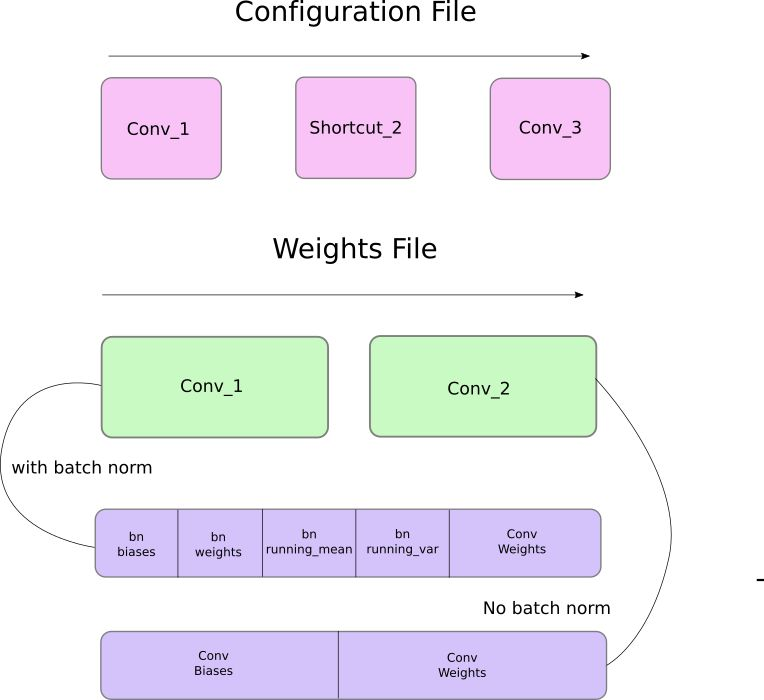def load_weights(self, weightfile):

 #Open the weights file fp = open(weightfile, "rb") #The first 5 values are header information  # 1. Major version number # 2. Minor Version Number # 3. Subversion number  # 4,5. Images seen by the network (during training) header = np.fromfile(fp, dtype = np.int32, count = 5) self.header = torch.from_numpy(header) self.seen = self.header

 weights = np.fromfile(fp, dtype = np.float32)

 ptr = 0 for i in range(len(self.module_list)): module_type = self.blocks[i + 1]["type"] #If module_type is convolutional load weights #Otherwise ignore.

 if module_type == "convolutional": model = self.module_list[i] try: batch_normalize = int(self.blocks[i+1]["batch_normalize"]) except: batch_normalize = 0 conv = model

 if (batch_normalize): bn = model #Get the number of weights of Batch Norm Layer num_bn_biases = bn.bias.numel() #Load the weights bn_biases = torch.from_numpy(weights[ptr:ptr + num_bn_biases]) ptr += num_bn_biases bn_weights = torch.from_numpy(weights[ptr: ptr + num_bn_biases]) ptr += num_bn_biases bn_running_mean = torch.from_numpy(weights[ptr: ptr + num_bn_biases]) ptr += num_bn_biases bn_running_var = torch.from_numpy(weights[ptr: ptr + num_bn_biases]) ptr += num_bn_biases #Cast the loaded weights into dims of model weights.  bn_biases = bn_biases.view_as(bn.bias.data) bn_weights = bn_weights.view_as(bn.weight.data) bn_running_mean = bn_running_mean.view_as(bn.running_mean) bn_running_var = bn_running_var.view_as(bn.running_var) #Copy the data to model bn.bias.data.copy_(bn_biases) bn.weight.data.copy_(bn_weights) bn.running_mean.copy_(bn_running_mean) bn.running_var.copy_(bn_running_var)

 else: #Number of biases num_biases = conv.bias.numel() #Load the weights conv_biases = torch.from_numpy(weights[ptr: ptr + num_biases]) ptr = ptr + num_biases #reshape the loaded weights according to the dims of the model weights conv_biases = conv_biases.view_as(conv.bias.data) #Finally copy the data conv.bias.data.copy_(conv_biases)

#Let us load the weights for the Convolutional layersnum_weights = conv.weight.numel()#Do the same as above for weightsconv_weights = torch.from_numpy(weights[ptr:ptr+num_weights])ptr = ptr + num_weightsconv_weights = conv_weights.view_as(conv.weight.data)conv.weight.data.copy_(conv_weights)

model = Darknet("cfg/yolov3.cfg")model.load_weights("yolov3.weights")`

0
0 收藏

### 作者的其它热门文章0 评论
0 收藏
0